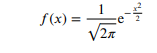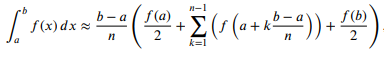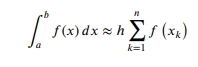# Check if I have done everything right

2 views (last 30 days)
Mathias Pettersen on 24 Sep 2019
Commented: Rena Berman on 28 Oct 2019
Hey, I am working on an assignement and I am wonder if you can check if what I've done so far is right.
Here are the questions:
1. Make a vector from -3 to 3 with 1000 elements with the use of linspace function
2. Standard function is given:Make a plot showing the function values for x [-3,3]. Use the vector from task 1.
3. Make a m. file implementing the function f(x) as a matlab function. Call this matlab function calcf and the .m file calcf.
4. Use the vector created in the first task to calculate the function values and plot the resault.
5. By sending a vector as an argument to your function, the function values are calculated in one step. Make a for loop calculating each function value one by one
store these in an empty vector m. Use the zeros function to make this vector m. Be careful that it has the right dimensions (E.G. 1 x 1000 for a row vector).
6. Trapez method can be used to find the value of the integral of a function numeric:Make a loop to calculate the function over. When f(x) is standard normal distributed like over, a = -3. b = 3 and n = 1.
7. An easier method is by using this formula:Here h = x(k+1) - x(k). X(k) means value number k in the set x. Which is the same as writing x(k). Here h is constant. Make an m-file implementing Riemann as a
matlab function. Call the function riemann and the m.file riemann.m. The function must take x and y = f(x) values as arguments. The function must return the value
of the numeric integral.
Here are my answers to these questions:
% 1)
x = linspace(-3,3,1000);
% 2)
f = (1/sqrt(2*pi)) * exp(-(x.^2)/2);
% 3) In the calcf.m
% 4)Call function calcf and plot it
calcf(x);
plot(x,f)
% 5) Zeros vector from 1:1000
y = zeros(size(calcf(x)));
for i = 1:max(size(calcf(x)))
y(i) = f(i)
end
% 6) Trapez
a = -3;
b = 3;
n = 10;
trapez = 0;
for k = 1: n - 1
trapez = trapez + sum(calcf(a + (k.*(b-a)./n)) + calcf(b)./2);
end
trapez = trapez + calcf(a)/2;
trapez = trapez * ((b-a)/n)
Here is my calcf function for task 3:
function [f] = calcf(x)
f = (1/sqrt(2*pi)) * exp(-(x.^2)/2);
end
Here is the riemann function which im currently having a hard time with. I know the input function x and y are not being used but I dont know how I should use them as task 7 is wanting me to.
function [integral] = riemann(x, y)
n = 10;
k = 1;
integral = (sum(calcf(k))).*n;
h = calcf(k+1) - calcf(k);
integral = integral * h;
end
I hope you can see if what I've done is right and I know some of it is definetly wrong but I cant seem to solve it.

#### 1 Comment

Rena Berman on 28 Oct 2019

Michal Kvasnicka on 24 Sep 2019
Edited: Michal Kvasnicka on 24 Sep 2019
Your riemann function is completely wrong!
Try this:
function [integral] = riemann(x)
integral = sum(calcf(x));
h = x(2) - x(1);
integral = integral * h;
end
But I am really not sure if you understand the Matlab basics, because your code is, frankly speaking, terrible.

Guillaume on 24 Sep 2019
Edited: Guillaume on 24 Sep 2019
2) you haven't done what's asked (plot the function)
4) is wrong (hint clear f and run that again)
5) you haven't done what's asked (pass scalars to the function)
6), 7), I'm not going to check. Have you checked that you get the proper result? Note that naming a variable integral is not a good idea (as it shadows the integral function)